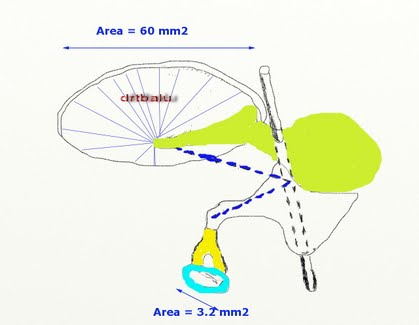# Impedance matching function of middle ear cavity

Impedance matching is one of the important functions of middle ear. The middle ear transfers the incoming vibration from the comparatively large, low impedance tympanic membrane to the much smaller, high impedance oval window.

Middle ear is an efficient impedance transformer. This will convert low pressure, high displacement vibrations into high pressure of the air into, low displacement vibrations suitable for driving cochlear fluids.

The impedance of cochlear fluids is approximately equal to that of sea water (i.e. 1.5 x 106 N.sec/m3 ). Because of this high impedance of cochlear fluids only 0.1 % of incident energy would be transmitted.

Two processes are involved in the impedance matching mechanism of middle ear. They are:

1. The area of the tympanic membrane is larger than that of the stapes foot plate in the cochlea. The forces collected over the ear drum are concentrated over a smaller area, thus increasing the pressure over oval window. The pressure is increased by the ratio of these two areas i.e. 18.75 times.

2. The second process is the lever action of the middle ear bones. The arm of the incus is shorter than that of the malleus, and this produces a lever action that increases the force and decreases the velocity at the stapes. Since the malleus is 2.1 times longer than the incus, the lever action multiplies the force by 2.1 times.Figure showing impedance matching function of middle ear